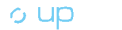Calculate Remaining Weeks of Benefits
To Calculate Remaining Weeks of Benefits:
• Find the column labeled "Status".
• In the "Status" column, locate a row labeled "Open" that has a remaining balance.
• Divide the amount listed under "Remaining Balance" by the number in that same row under the "Weekly Benefits" column.
• The result is the number of weeks of benefits you have left.
Using the example above, the calculation should look like this: Remaining Balance (\$2,808.00) / Weekly Benefit (\$108.00) = Weeks of Benefits Remaining (26)